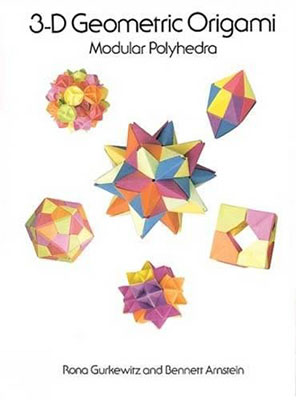This image was scanned from my private collection TABLE OF CONTENTS Introduction Part One: Background Material What is a Polyhedron Illustrations and Facts about Polyhedra      Platonic Solids      Archimedean Solids      Kepler-Poinsot Solids      Miscellaneous Solids Polyhedra: Connections with Different Fields Part Two: Making Models Using the Diagrams Definition of Symbols The Assembly Process Creating Your Own Models Part Three: Preliminary Constructions Equilateral Triangles      How to Make Equilateral Triangles      How to Make 20 Equilateral Triangles from a Square or 28 Equilateral Triangles from an 8 1/2" x 11" Rectangle      Equilateral Triangle Tessellation for Snub Cube Flat Pattern      Equilateral Triangle Tessellation for Snub Dodecahedron Flat Pattern Pentagons      How to Fold a Pentagon from a Square      Pentagon Template      How to Use the Pentagon Template Hexagons      How to Make Hexagons      How to Fold a Square or Rectangle into Three Equal Parts Part Four: Diagrams of Models Simple Construction to Try First      Inflatable Irregular Octahedron      Puzzle Cube      Tetrahedron from Siamese-Twin Hexagons      Two-Piece Octahedron Skeleton      Truncated Tetrahedron from Hexagons      Eight-Sided Module: Truncated Cube Flat Unit System      Square Module Flat Unit: Small Rhombicuboctahedron      Hexagon Module from a Triangle      Triangle Module from a Hexagon One-Piece Module System      One-Piece Triangle Module      One-Piece Square Module Simple Dodecahedron Systems      Dodecahedron Flower Ball      Modular Dimpled Dodecahedron Ball Cuboctahedron Systems      Spike Ball Module      Spike Ball and Super Spike Ball      Ornamental Ball Module      Simple Square Module      Three-Loop Cuboctahedron Equilateral Triangle Module Systems      Equilateral Triangle Strip System I      Equilateral Triangle Strip System II      10-Module Icosahedron from Equilateral Triangle Strip System I and II      Miscellaneous Models      Triangle Edge Module Stellation Module Systems      36-Degree Isosceles Triangle Module      45-Degree Isosceles Stellation Module Great Dodecahedron Systems      Pentagon Module: Great Dodecahedron      Simplified Pentagon Module: Great Dodecahedron      12-Module Great Dodecahedron Flat Patterns from Equilateral Triangle Tessellations      Flat Patterns That Can Be Laid Out on an Equilateral Triangle Tessellation      Truncated Hexadecahedron Flat Pattern      Snub Cube and Snub Dodecahedron Flat Patterns Supplementary Material Finding Models in the Book: Index by Polyhedron Books of Related Interest Finding Other Folders About the Authors Title: 3-D Geometric Origami: Modular Polyhedra Author: Bennett Arnstein, Rona Gurkewitz Format/Publication Date: TPB:1995 Publisher: Dover Publications, Inc., Mineola, NY Language: English Page Count: 73 Book Dimensions(ht. x w.): 12" x 9" ISBN: 0486288633 SUMMARY- This book is a lot more accessible to a novice than some of the other books I've looked at, with enough else going on to be of interest to someone more advanced. There's a nice shout out to the pioneers in this field of origami in Rona's introduction.